# The QUANTSELECT Procedure (Experimental)

### Example 78.1 Simulated Data Example

This simulation study exemplifies the unity of motive and effect for the PROC QUANTSELECT procedure. The following statements generate a data set that is based on a naive instrumental model (Chernozhukov and Hansen, 2008):

%let seed=321;
%let p=20;
%let n=3000;

data analysisData;
array x{&p} x1-x&p;
do i=1 to &n;
U  = ranuni(&seed);
x1 = ranuni(&seed);
x2 = ranexp(&seed);
x3 = abs(rannor(&seed));
y  = x1*(U-0.1) + x2*(U*U-0.25) + x3*(exp(U)-exp(0.9));
do j=4 to &p;
x{j} = ranuni(&seed);
end;
output;
end;
run;


Variable U of the data set indicates the true quantile level of the response y conditional on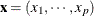.

Let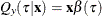denote the underlying quantile regression model, where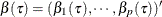. Then, the true parameter functions are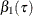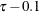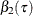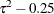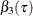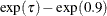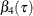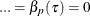It is easy to see that, at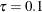, only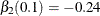and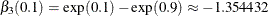are nonzero parameters. Therefore, an effective effect selection method should select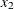and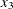and drop all the other effects in this data set at. By the same rationale,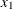andshould be selected at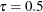with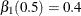and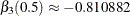, andandshould be selected at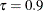with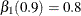and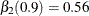.

The following statements use PROC QUANTSELECT with the adaptive LASSO method:

proc quantselect data=analysisData;
model y= x1-x&p / quantile=0.1 0.5 0.9
run;


The selected effects and the relevant estimates are shown in Output 78.1.1 for, Output 78.1.2 for, and Output 78.1.3 for. You can see that the adaptive LASSO method correctly selects active effects for all three quantile levels.

Output 78.1.1: Parameter Estimates atThe QUANTSELECT Procedure

Quantile = 0.1

Selected Effects: Intercept x2 x3

Parameter Estimates
Parameter DF Estimate Standardized
Estimate
Intercept 1 0.011793 0
x2 1 -0.228709 -0.218287
x3 1 -1.379907 -0.784520

Output 78.1.2: Parameter Estimates atThe QUANTSELECT Procedure

Quantile = 0.5

Selected Effects: Intercept x1 x3

Parameter Estimates
Parameter DF Estimate Standardized
Estimate
Intercept 1 0.011778 0
x1 1 0.425843 0.118792
x3 1 -0.863316 -0.490822

Output 78.1.3: Parameter Estimates atThe QUANTSELECT Procedure

Quantile = 0.9

Selected Effects: Intercept x1 x2

Parameter Estimates
Parameter DF Estimate Standardized
Estimate
Intercept 1 -0.007738 0
x1 1 0.782942 0.218407
x2 1 0.576445 0.550177

The QUANTSELECT procedure can perform effect selection not only at a single quantile level but also for the entire quantile process. You can specify the QUANTILE=PROCESS option to do effect selection for the entire quantile process. With the QUANTILE=PROCESS option specified, the ParameterEstimates table produced by the QUANTSELECT procedure actually shows the mean prediction model of y conditional on. In this simulation study, the true mean model is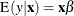where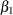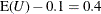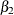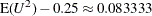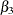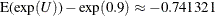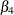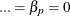The following statements perform effect selection for the quantile process with the forward selection method.

proc quantselect data=analysisData;
model y= x1-x&p / quantile=process(ntau=all)
selection=forward;
run;


Output 78.1.4 shows that, by default, the SELECT= and STOP= options are both set to SBC. The selected effects and the relevant estimates for the conditional mean model are shown in Output 78.1.5.

Output 78.1.4: Model Information

The QUANTSELECT Procedure

Model Information
Data Set WORK.ANALYSISDATA
Dependent Variable y
Selection Method Forward
Quantile Type Process
Select Criterion SBC
Stop Criterion SBC
Choose Criterion SBC

Output 78.1.5: Parameter Estimates

Parameter Estimates
Parameter DF Estimate Standardized
Estimate
Intercept 1 0.007833 0
x1 1 0.418825 0.116834
x2 1 0.094791 0.090472
x3 1 -0.785686 -0.446687

Linear regression is the most popular method for estimating conditional means. The following statements show how to select effects with the GLMSELECT procedure, and Output 78.1.6 shows the resulting selected effects and their estimates. You can see that the mean estimates from the QUANTSELECT procedure are similar to those from the GLMSELECT procedure. However, quantile regression can provide detailed distribution information, which is not available from linear regression.

proc glmselect data=analysisData;
model y= x1-x3 / selection=forward(select=sbc stop=sbc choose=sbc);
run;


Output 78.1.6: Parameter Estimates

The GLMSELECT Procedure
Selected Model

Parameter Estimates
Parameter DF Estimate Standard Error t Value
Intercept 1 -0.010143 0.043129 -0.24
x1 1 0.434553 0.057385 7.57
x2 1 0.114183 0.016771 6.81
x3 1 -0.797194 0.028156 -28.31